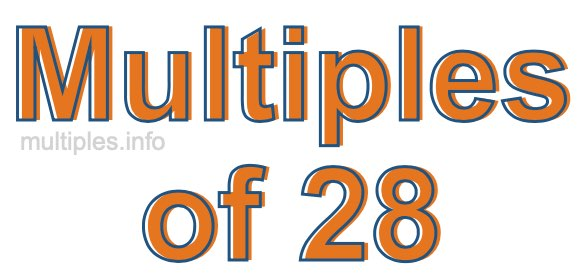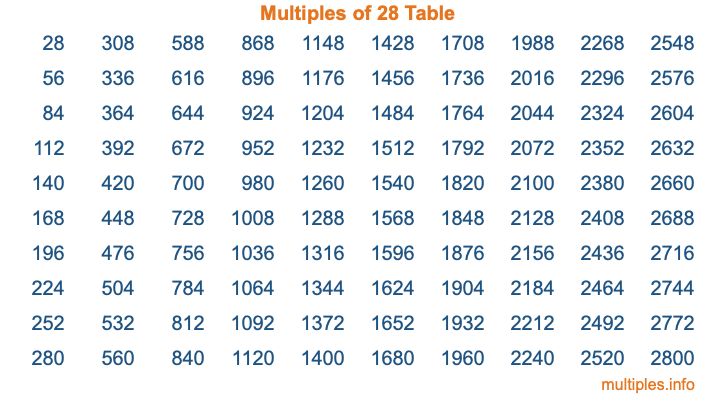Multiples of 28Welcome to the Multiples of 28 page. Here we will first teach you everything you will ever need to know about the multiples of 28, and then give you a study guide summary of everything we taught you to make sure you remember it all. Use this page to look up facts and learn information about the multiples of 28. This page will make you a multiples of twenty-eight expert!

Definition of Multiples of 28
Multiples of 28 are all the numbers that when divided by 28 equal an integer. Each of the multiples of 28 are called a multiple. A multiple of 28 is created by multiplying 28 by an integer.

Therefore, to create a list of multiples of 28, you start with 1 multiplied by 28, then 2 multiplied by 28, then 3 multiplied by 28, and so on for as long as you want. Thus, the list of the first five multiples of 28 is 28, 56, 84, 112, and 140. To see a larger list of multiples of 28, see the printable image of Multiples of 28 further down on this page. We also have a category where you can choose any nth multiple of 28.

Multiples of 28 Checker
The Multiples of 28 Checker below checks to see if any number of your choice is a multiple of 28. In other words, it checks to see if there is any number (integer) that when multiplied by 28 will equal your number. To do that, we divide your number by 28. If the the quotient is an integer, then your number is a multiple of 28.

Is  a multiple of 28?

Least Common Multiple of 28 and ...
A Least Common Multiple (LCM) is the lowest multiple that two or more numbers have in common. This is also called the smallest common multiple or lowest common multiple and is useful to know when you are adding our subtracting fractions. Enter one or more numbers below (28 is already entered) to find the LCM.

Check out our LCM Calculator if you need more details about the Least Common Multiple or if you need the LCM for different numbers for adding and subtraction fractions.

nth Multiple of 28
As we stated above, 28 is the first multiple of 28, 56 is the second multiple of 28, 84 is the third multiple of 28, and so on. Enter a number below to find the nth multiple of 28.

th multiple of 28

Multiples of 28 vs Factors of 28
28 is a multiple of 28 and a factor of 28, but that is where the similarities end. All postive multiples of 28 are 28 or greater than 28. All positive factors of 28 are 28 or less than 28.

Below is the beginning list of multiples of 28 and the factors of 28 so you can compare:

Multiples of 28: 28, 56, 84, 112, 140, etc.

Factors of 28: 1, 2, 4, 7, 14, 28

As you can see, the multiples of 28 are all the numbers that you can divide by 28 to get a whole number. The factors of 28, on the other hand, are all the whole numbers that you can multiply by another whole number to get 28.

It's also interesting to note that if a number (x) is a factor of 28, then 28 will also be a multiple of that number (x).

Multiples of 28 vs Divisors of 28
The divisors of 28 are all the integers that 28 can be divided by evenly. Below is a list of the divisors of 28.

Divisors of 28: 1, 2, 4, 7, 14, 28

The interesting thing to note here is that if you take any multiple of 28 and divide it by a divisor of 28, you will see that the quotient is an integer.

Multiples of 28 Table
Below is an image of the first 100 multiples of 28 in a table. The table is in chronological order, column by column. The first column has the first ten multiples of 28, the second column has the next ten multiples of 28, and so on.The Multiples of 28 Table is also referred to as the 28 Times Table or Times Table of 28. You are welcome to print out our table for your studies.

Negative Multiples of 28
Although not often discussed or needed in math, it is worth mentioning that you can make a list of negative multiples of 28 by multiplying 28 by -1, then by -2, then by -3, and so on, to get the following list of negative multiples of 28:

-28, -56, -84, -112, -140, etc.

Multiples of 28 Summary
Below is a summary of important Multiples of 28 facts that we have discussed on this page. To retain the knowledge on this page, we recommend that you read through the summary and explain to yourself or a study partner why they hold true.

There are an infinite number of multiples of 28.

A multiple of 28 divided by 28 will equal a whole number.

28 divided by a factor of 28 equals a divisor of 28.

The nth multiple of 28 is n times 28.

The largest factor of 28 is equal to the first positive multiple of 28.

28 is a multiple of every factor of 28.

28 is a multiple of 28.

A multiple of 28 divided by a divisor of 28 equals an integer.

28 divided by a divisor of 28 equals a factor of 28.

Any integer times 28 will equal a multiple of 28.

Multiples of a Number
Here you can get the multiples of another number, all with the same attention to detail as we did for multiples of 28 on this page.

Multiples of
Multiples of 29
Did you find our page about multiples of twenty-eight educational? Do you want more knowledge? Check out the multiples of the next number on our list!